### Deterring Imperfectly Informed Tortfeasors: Optimal Rules for Penalty and Liability

by David D. Friedman

Part I: Introduction

An individual T considers an action which will produce a gain g to him but a loss l to someone else. If g>l the action produces a net gain of g-l and, by the usual criterion of economic efficiency, ought to be taken. If g<l it produces a net loss of l-g and ought not to be taken. The problem facing the designer of an optimal legal system is how to make it in T's interest to act in that way. A familiar solution is to impose a penalty f on T equal to l. Since his private net gain is now equal to the social net gain, he will take the action if and only if it is efficient to do so.

So far I have assumed that T, when he takes the action, knows l with certainty. Suppose instead that he knows only a probability distribution [[rho]](l). Further assume, for simplicity, that T is risk neutral and that the penalty can be imposed with no net cost.

The rule for maximizing the social benefit is now to take the action if the expected net gain g - <l> is positive, where

<l> =[[integral]] l[[rho]](l)dl

One way to produce that result is to impose a penalty f on T equal to whatever the value of l turns out to be. His expected penalty is then:

<f> = [[integral]] f[[rho]](l)dl = [[integral]] l[[rho]](l)dl =<l>

His (private) expected net gain is <g-f> = g - <f> = g - <l>

So private expected net gain is equal to social expected net gain, and it is again in the private interest of T to act efficiently.

The argument so far seems obvious, even trivial. But the conclusion-that we produce optimal behavior ex ante by imposing on each offender a penalty equal to the actual loss he causes ex post-is inconsistent with the conclusion that Polinsky reached in his analysis of essentially the same problem. In his 1987 article he concluded that, when the injurer's information about the victim's loss is imperfect, the optimal rule is to impose a punishment l+d, where l is the actual loss. He argued that d should be negative (penalty less than damage done) for "socially desirable" actions-ones where g is usually > l-and that d should be positive for "socially undesirable" actions-ones where g is usually < l.

The purpose of this article is to explore the conflict between the argument for punishment equal to damage done and the argument given by Polinsky for punishment not equal to damage done. In doing so, I will show that Polinsky's argument depends on the assumption that the people writing the legal rules have access to information that potential offenders do not have and cannot be given.

In Part II, I sketch Polinsky's argument and suggest some difficulties with it. In Part III, I work through the analysis for a particular example, analysing the implications of both the assumptions Polinsky makes and the assumptions that I argue he ought to make. In Part IV I analyse the general case, showing that penalty equal to damage done gives the best possible result, assuming that potential offenders have access to the same information (ex ante) as the legal system and use it rationally. One implication of the argument is that the court, in Polinsky's situation, ought to publish its information, along with an announcement that it will set the penalty equal to the damage done. By doing so it will do at least as well as if it follows Polinsky's prescription. In Part V, I briefly discuss the application of the analysis to the choice between strict liability and negligence rules. Finally, in Part VI, I consider the effect of including punishment cost in the analysis.

Part II: Polinsky's Argument

An individual T is deciding whether to take an action that produces a gain g for him and a loss l for someone else. T does not know the actual value of l. He draws a value l' from a a distribution h(l'), and treats it as if it were the actual value of l. If he knows that he will be liable for a damage payment equal to the damage done, he will take the action if g>l' but not if g<l'.

Polinsky writes:

"...it will be demonstrated that the optimal level of liability generally is not equal to the victim's loss. Whether liability should be above or below the loss depends on the social desirability of the activity-that is, on whether the average gain of injurers is less than or greater than the victim's loss. To see why, consider the rule of strict liability and suppose that the court makes the injurer liable exactly for the victim's loss. ... If an injurer's estimate of the loss is less than the true loss, that injurer might be underdeterred-that is, he might engage in the activity even though his gain is less than the victim's loss. But if an injurer's estimate of the loss is above the true loss, the injurer might be overdeterred-he might not engage in the activity even though his gain exceeds the victim's loss. Thus, using compensatory damages leads to two possible errors.

Suppose instead that the court adjusts compensatory damages upward by some amount. Assuming that injurers then raise their estimates of their liability, this adjustment decreases the number of injurers who will be underdeterred but increases the number who will be overdeterred. If the gains of most injurers are below the victim's loss-that is, if the activity is socially undesirable-then the imposition of additional damages results in a net social benefit. Conversely, if the activity is socially desirable, it is optimal to impose less than compensatory damages on injurers since the benefit from reducing overdeterrence is more important than the detriment from increasing underdeterrence."

In other words, if whoever is setting up the legal system knows that the action is "socially desirable" (the probability that g>l is large) then he knows that most T's ought to take the action, so situations in which T should not take the action but does (is underdeterred), due to his misestimate of l, will be rare relative to situations in which he should take the action but does not. So the first source of inefficiency is likely to produce much larger costs than the second. So an announcement that tortfeasors will be charged less than the damage done (d<0) should produce a net improvement.

One can see the argument most clearly by considering a case where g>l for all potential tortfeasors but some overestimate loss by enough to make l'>g. There is nobody we want to deter, so lowering the damage rule provides a benefit (some tortfeasors are no longer deterred) but no cost.

This example suggests one problem with the argument. I have assumed, following Polinsky, that T believes that l' is the damage he will inflict if he takes the action. But if everyone knows that the particular action is always efficient-that g>l for all T-then a particular T who gets a value of l'>g will know it is wrong and ignore it. He cannot be someone for whom the action is inefficient, because there are no such people.

More generally, if the individual actors have access to the same information as whoever is setting up the legal system, they ought to use that information in making their decision. Yet Polinsky assumes that individuals accept their estimate l' as the true value of l, whatever the actual distribution of gains and losses. In the limiting case where the act is always efficient, his assumptions amount to assuming that the tortfeasor will believe his l' even though it is impossible.

Polinsky deals with this problem by assuming that "the court has better information about the loss than the injurer." One objection to that assumption is that it can be used to produce very nearly any result one wants. We could, for example, assume that the court system knows that actors, on average, overestimate (or underestimate) the damage they will do, and that the damage rule should be adjusted accordingly. A second objection is that such an assumption leaves us wondering why, if the information can be used to produce better choices by the actors, the court system does not simply publish it.

A further objection is that the assumption of superior knowledge by the court system undercuts the argument for making the announced damage rule depend on the actual damage done, with or without an adjustment. If we take Polinsky's model literally, the court could eliminate both kinds of inefficiency by simply announcing that the punishment for the offense is a fixed penalty of l, what the court knows the loss will be. Individuals would then ignore their estimate l' and make their decision according to whether or not g>l (l having been announced by the court), which is the efficient rule.

One could eliminate this simple solution by representing the loss l by a probability distribution rather than a single value. But that does not eliminate the essential problem. By announcing that the punishment depends on the damage actually done, the court gives individuals an incentive to base their decision on their estimate of the damage. There is no reason the court should want them to do so unless the individual actors have information about the damage they are doing that the court does not also have.

In order to understand and deal with such problems, it will be necessary to specify more clearly what the court system and the actors know and how they use the information. In part III I will do so for a specific distribution of (l,l'), and in Part IV for the general case. In part V I will discuss the implications of the analysis for the choice between strict liability and negligence, and in VI the complications introduced by taking account of the cost of imposing damages on tortfeasors.

Part III: A Simple Case

Let l and l' both be uncertain; their joint distribution, known to both the court and the actors, is h(l,l'). The individual actor T receives a gain g which is, for the moment, assumed to be the same for all T.

In Part IV, I will provide a formal treatment of the general problem. In this part, I will work through the analysis for a particular example. In doing so, I will show that Polinsky's conclusion is correct under his assumptions but incorrect if actors have the same information about the distribution of gains and losses as the court system. I will conclude by comparing the outcome implied by Polinsky's assumptions (actor takes l' as l, court system adjusts the damage rule optimally) to the outcome implied by my assumptions (actor estimates l using l' and h(l,l'), court system sets the penalty equal to l).

We start by assuming h(l,l') uniform over 1<l<2, l-.5<l'<l+.5. On Figure 1a, h(l,l') = 1 on the shaded region and 0 elsewhere. Figure 1b shows the distribution of l' for a fixed value of l (l = 1.8). Since h(1.8,l') is uniform from 1.8-.5 to 1.8+.5, the expected value of l' is <l'>=1.8=l. So this distribution is unbiased in the sense used by Polinsky.Figure 1

Figure 2b shows h(l,2)-the distribution of l for a fixed value of l' (l'=2). This is the probability distribution for l from the standpoint of an individual T who knows that l'=2. In Polinsky's model, T simply assumes that l=l'=2, and decides whether to commit the offense according to whether the gain is more or less than 2. Figure 2b shows why this is wrong for an individual who (like the court system formulating the legal rules) knows the form of h(l,l'). l is uniformly distributed from 1.5 to 2, so its expected value is <l>=1.75 .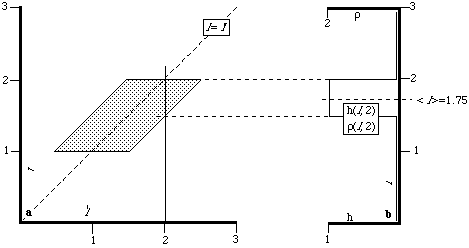Figure 2

This example demonstrates that while the distribution is unbiased, the rule Polinsky's actor follows is not. For a high value of l' Polinsky's rule (assume l=l') yields an estimate of l higher than its expected value conditional on that l'. Similarly, for a low value of l', the rule yields an estimate of l lower than its expected value. If g is high ("socially desirable act") then the marginal actors are ones for whom l' is high. By following Polinsky's rule they overestimate l. By telling them that the damages they will owe will be less than l (d<0), the court compensates for that overestimate. Similarly, with a low g ("socially undesirable act") the court can compensate by setting d>0. Thus Polinsky's conclusion, that punishment should be set higher than damage done for socially undesirable acts and lower for socially desirable acts, is the result of an assumed bias in the actors' estimates-a bias not in the distribution of l' but in the estimate of l.

The argument can be made more precise by actually solving for the value of d that maximizes net benefit for the distribution h(l,l') shown in the figures. I will do so twice-once for an actor Tp following Polinsky's rule and once for a rational actor Tr who knows h(l,l') and combines that information with his value of l' in calculating the distribution of l.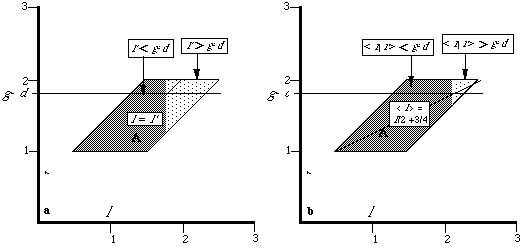Figure 3 Figure 4

Situation 1: Polinsky's Actor

We assume the court announces that the punishment will be f=l+d. A potential tortfeasor Tp commits the act if his gain g is greater than his estimate of l+d. Net benefit is then g-l, averaged over the region of (l,l') for which Tp would commit the act. So we have:

Net Benefit = NB(d) =where A is the region containing all values of (l, l') such that Tp would commit the act.

We assume, with Polinsky, that Tp assumes l=l', so A is defined by:

l'+d<g

as shown on Figure 3, and we have:

If g-d<1.5 then (case 1)

NB(d) =At d*, the value of d that maximizes NB, we have: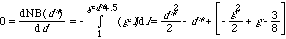Solving for d* and choosing the root for which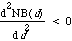yields: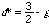If g-d>1.5 then (case 2)

NB(d)Solving for d*, and choosing the root for which, again yields:At g-d=1.5,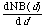is the same from both sides; we do not have to worry about an additional maximum at the boundary between the regions corresponding to cases 1 and 2. So the value of d that maximizes net benefit under Polinsky's assumptions is. If g>3/2 the act is socially desirable, in Polinsky's sense, and d*<0; the court announces a penalty of less than the damage done. Similarly, if g<3/2, the act is socially undesirable, d*>0, and the penalty is more than the damage done. So the formal analysis of this particular example gives the result we would expect from Polinsky's arguments.

Situation 2: A Rational and Informed Actor

What happens if, instead of requiring T to assume that l=l', we let him use l' and h(l,l') to estimate l? The region A, the set of values of (l,l') for which the rational actor Tr chooses to act, is now defined by:

<l>+d < g

If l'>3/2 then l is uniformly distributed between l'-1/2 and 2 , so <l> =. If l'<3/2, then l is uniformly distributed between 1 and l'+1/2, so <l> =So <l>as shown on Figure 4. The region A is then defined by: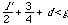and we have:

If 2(g-d-3/4) <1.5 (case 1) then

NB(d) =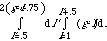At d*, the value of d that maximizes NB, we have: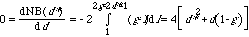Solving for d*, and choosing the root for which, yields:

d*=0

If 2(g-d-3/4) >1.5 then (case 2)

NB(d)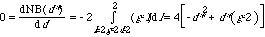Solving for d*, and choosing the root for which, again yields:

d*=0

At 2(g-d-3/4)=1.5,is again the same from both sides, so again we do not have to worry about an additional maximum at the boundary between the two regions. It follows that d*=0. Whatever the value of g, the optimal rule is to set the penalty equal to the damage done.

We have shown that, for Tp, the optimal damage rule involves a penalty that is not in general equal to l, with the difference depending on g, while for Tr the optimal rule is to set the penalty equal to the loss l. This suggests a further question: which situation provides a higher net benefit? Are we better off having actors who follow Polinsky's rule of treating l' as if it were l and a court system that compensates for their behavior by appropriately adjusting the damage rule, or are we better off with actors who rationally calculate the distribution of l from l' and h(l,l') and a court system that sets punishment equal to damage done?

The answer is that, under our present assumptions, the two situations are equally attractive-indeed, they produce precisely the same results. In either situation, there is a critical value of l', which I will call, such that individuals for whom l'>are deterred and do not take the action, while individuals for whom l'<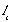are not deterred and do take the action.

In the case of Tp, we have:

Estimated private benefit from the action = g-l'-d

Tp takes the action if he believes that private benefit is positive. The court sets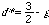. So we have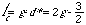(Equation 1)

In the case of Tr we have:

<l>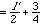Estimated private benefit = g-<l>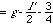Tr takes the action if he believes that private benefit is positive, so we have: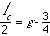(Equation 2)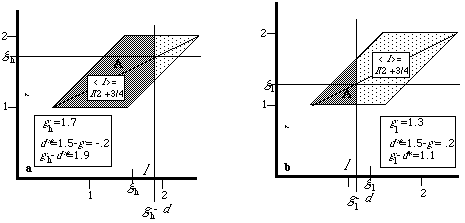Figure 5

Equations 1 and 2 are identical; the two rules lead to exactly the same behavior and thus the same net benefit. Figure 5 illustrates the result for two values of g. Each results in a different value of d*; in each case the region A for which the actor commits the action is the same as it would be with a rational actor Tr.

One advantage of a tort system, or more generally of any system of pigouvian taxes, is that it lets the actor incorporate his private information about his own costs and benefits into his decision. Just as on an ordinary market, he is faced with a price, representing the cost his action imposes on others, and left free to decide whether the benefit to him of taking an action is worth paying that price. Under such a system the court need not know how much the action is worth to the actor in order to induce him to make the efficient decision. So far, however, I have assumed that the gain g is the same for all actors and thus assumed away one of the reasons for having a tort system. The next step is to drop that assumption and see what the effect is on our conclusions.

Assume that g is different for different actors and that the actor knows his value of g but the court does not. This change in our assumptions has no effect on the analysis of Tr; the optimal damage rule does not depend on g, so the court does not need to know g in order to calculate it. But the damage rule for Tp depends on g. If d is given the value that is optimal for one value of g, it will be too high or too low for another. So net benefit will be lower than it would be if d could be set at its optimal value for each different g. We have just shown that Tr, faced with a penalty equal to damage done, behaves just as Tp would if faced with the optimal penalty l+d*. So Tr , faced with a penalty equal to l, will do better than a Tp faced with a suboptimal penalty.

It follows that, if g varies, the situation described by Polinsky produces a lower net benefit than a situation in which the actor is aware of h(l,l') and rationally combines the information with his value of l' in deciding whether to take the action. That implies that if the court knows h(l,l') and the actors do not, the court should publish h(l,l') and announce that the penalty will be equal to the damage done. The result is a greater net benefit than if the court kept the information to itself and announced a penalty equal to l+d.

Part IV: The General Case

We assume that the individual, like the court system, knows g and h(l,l'). In addition, he knows (and the court does not) the value of l' that he has drawn. His probability distribution for l given l' is, by Bayes' theorem: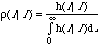If the damage rule is damages=l+d, then, from the standpoint of the individual actor,

<Damages>=<l+d>=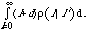= d +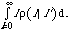= d +But the expected loss is

<l> ==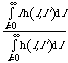Since the efficient rule (ex ante) is to take the action if and only if g is greater than <l>, the optimal value of d is 0. We have simply repeated the analysis we started with, using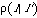instead of [[rho]](l). Sincewas calculated using all of the available information, it provides the best available measure of <l>.

A more formal way of putting the argument is to start by noting that, since the only information one actor has that distinguishes him from another is his value of l', any damage rule is equivalent to a rule specifying for which values of l' an actor will or will not take the action. Net benefit is: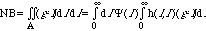where A is the set of values of (l,l') for which Tr chooses to take the action, and (equivalently)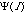=1 for values of l' for which Tr takes the action and 0 otherwise. So we maximize NB with a rule such that=1 (Tr takes the action) if>0 (Inequality 3)

and=0 (Tr does not take the action) if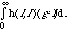<0.

But the individual Tr with a particular value of l' commits the act if:

Expected gain =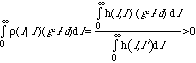(Inequality 4)

h(l,l') > 0 for all values of l,l', since it is a probability density, so the denominator is positive. It follows that, if d=0, Inequality 4 is equivalent to Inequality 3: d=0 results in behavior that maximizes net benefit.

Part V: Strict Liability vs Negligence

So far we have assumed strict liability: Tr must pay damages whether or not g>l. It is interesting to ask, as Polinsky does, how the assumption that the actor has imperfect information about l affects the relative efficiency of strict liability and negligence.

The answer will depend on how negligence is defined. If the court holds that an actor is negligent if and only if the expected loss, calculated from the information available to the actor when he acted, is greater than the actor's gain, and if the court has the information necessary to determine negligence on that basis, then negligence and strict liability will lead to the same behavior for Tr and so be equally efficient. The only effect of shifting to negligence is that, in situations where Tr would have taken the action and paid damages, he now takes the same action but does not have to pay damages.

It seems more plausible, however, to assume that some of the information used by an individual deciding whether to commit a tort is private information to which the court has no access. In the context of our model, we may represent this information as l', the actor's information about the damage that his act will do. h(l,l') is then public information available to both the actor and the court, while l, the actual loss, becomes public information after the tort occurs but before the court sets damages.

Under these circumstances, the court can define negligence either in terms of the actual loss l or the expected loss <l> calculated from h(l,l') without any information on l'. I will assume that it does the former; the alternative assumption leads to similar results.

If we use a negligence rule and set the penalty equal to damage done, and if we assume that the act will be considered negligent if l>g, the result is inferior to strict liability. Under negligence, the penalty is the same as under strict liability if g<l and 0 if g>l. The average penalty, as calculated by Tr , is an integral over a range of l. As long as the range includes any values for which l<g, the average penalty will be lower under negligence. Since we have already proved that strict liability produces the best possible result (given our information), negligence must produce a worse result.

Could we solve this problem by setting a penalty higher than damage done? Consider the particular h(l,l') analysed in Part III. Under a negligence rule, the expected return of the act to a Tr for whom l'>3/2 is:The efficient result, as we have already seen, is (Equation 2):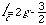Tr will commit the act if his gain is >0, so we have: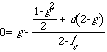(Equation 5)

Solving forand combining Equation 5 with Equation 2, we have: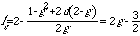Solving for d we get:(Equation 6)

Equation 6 gives us the value of d necessary to compensate for the fact that, under a negligence rule, the actor who happens to impose a loss less than his gain will not be punished. It depends on g. So if different actors have different values of g, there is no value of d that the court can announce which will reproduce the (efficient) incentives of a strict liability rule.

It follows that negligence is inferior to strict liability for actors who have imperfect information about the damage their acts will do, provided, as I have assumed, that the court does not have the information necessary to determine negligence on the basis of the offender's ex ante expected value for damage.

I have been assuming, for purposes of simplicity, that only the amount and not the occurrence of the loss is uncertain. Courts concerned with negligence are usually dealing with situations in which the effect of a precaution is not to reduce probability of loss from 1 to 0 but to change it by some small amount-perhaps from .001 to .0005. In applying the Hand formula, such a court will attempt to determine whether expected gain from precautions is more or less than expected cost.

The argument, however, still holds. Under strict liability, the potential tortfeasor, in deciding what to do, compares his gain to the expected loss (equal to his expected punishment) calculated from his ex ante probabilities. Under negligence, he does the calculation using both his probabilities and his estimate of what the court, ex post, will believe the ex ante probabilities to have been. His estimate of his expected punishment will be lower than under strict liability, because l is replaced by 0 a fraction pe of the time, where pe is the probability that the court will erroneously conclude that his gain was larger than his expected loss, making him not negligent and hence not liable. This will be true as long as the potential tortfeasor believes that the court's information about his ex ante situation is imperfect enough so that, even when he is in fact (ex ante) negligent, there is a non-zero chance that the court will think he is not.

Part VI: Uncertain Damage With Costly Punishment

So far I have assumed that imposing a punishment f is costless. Dropping that assumption will alter my conclusions in several ways. With costly punishment it is no longer optimal to make punishment equal to damage done, even if the damage that will be done is known with certainty. Combining uncertain damage with costly punishment introduces additional complications.

Costly Punishment With Certain Damage

Suppose the cost of imposing a punishment f on a single actor is C(f). Further suppose that the act imposes a certain loss l and that there is a distribution of gains g with a probability density m(g) and a cumulative probability density M(g), representing the probability that an actor will have a gain of at least g:

M(g) =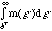.

The court system sets the punishment f to maximize net benefit, taking account of the punishment cost C(f), so it maximizes:

NB=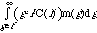by setting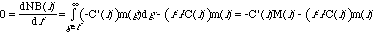(Equation 7)

The integral runs from g=f because only actors whose gain is at least equal to the punishment will take the action.

It is easy to see that NB is maximized at f=l only if

-C'(l)M(l)+C(l)m(l)=0. (Equation 8)

Equation 8 is equivalent to:C(f)M(f) is the total cost of punishment: punishment cost per offender times number of offenders. If it is independent of the level of punishment, then we are back with our old rule: set punishment equal to damage done.

Suppose instead that total punishment cost increases with the level of punishment-the increase in cost per offense as a result of imposing a more severe punishment outweighs the decrease in number of offenses. In that case deterring a slightly inefficient offense, an offense for which the net cost l-g is positive but small, may not be worth the cost of the increase in f necessary to deter it. If, on the other hand, total punishment cost decreases as level of punishment increases, we may want to deter even some offenses for which there is a small net gain: g- l positive but small. By deterring such an offense we save the cost of punishing it. Formally, we may rewrite Equation 7 as:If total punishment cost is increasing in the relevant range, the left hand side of the equation is negative, so the optimal punishment f is <l; if total punishment cost is decreasing the optimal fine is >l.

Costly Punishment with Uncertain Damage

Now suppose, as in earlier parts, that loss and information about loss are represented by a distribution h(l,l'). The assumption of costly punishment complicates our analysis in three different ways.

First, assume, as in much of our earlier analysis, that everyone has the same value of g. Setting punishment f = l is no longer optimal, for the reason we have just discussed in the context of certain damage. A lower punishment will reduce the punishment cost per offender but increase the number of offenders. If the net result is that lowering punishment lowers total punishment cost, then it may be worth failing to deter some offenses with net loss l-g>0, in order to save on punishment costs. If increasing punishment lowers total punishment cost, it may be worth deterring some offenses with net gain g-l>0. The correct rule is no longer f=l; the optimal schedule of punishments depends on the form of C(f) and the distribution h(l,l').

Second, assume a distribution of g, l and l'. Let h(l,l',g) be the joint distribution, where the actor knows g and l' when he decides whether to take the action, and the court knows l when it decides on the punishment. Our conclusions are now further complicated by the possibility that g may be related to l and l'. It might turn out, for example, that offenders with a value of l' implying a significant probability of a high value of l also had very high g, with the result that no punishment available to the court was sufficient to deter them. If so, we would want f(l) to be small, perhaps 0, for very high values of l-both because we would not want to deter those offenders (for most of them g>l) and because we could not deter most of them if we did want to.

More generally, the supply of offenses will have different elasticities with regard to punishments imposed on different values of l, and the elasticity with regard to f(l) at one value of l will depend on the values of f(l) at all other values, since a potential offender is facing an expected punishment that is a weighted average of f(l) over all values of l consistent with his value of l'. Formally we may write:(Equation 9)

whereNote that NB(f(l)) is not a function depending on l through f(l) but a functionel, mapping the function f(l) to a number NB(f(l)). If one had explicit forms for h(l,l',g) and C(f), one could use the calculus of variations to solve for the function f(l) that maximizes NB(f(l)).

Third and finally, punishment cost introduces another and unrelated complication. In our previous analysis, all that mattered about a punishment rule was the expected punishment <f> as calculated by an actor. Once we allow for punishment costs, two different patterns of punishment may have the same expected value for a particular actor but different costs.

Suppose, for example, that the marginal cost of punishment is increasing: C''(f)>0. Figure 6 shows such a C(f). Further suppose that offenders differ in g but not in the probability distribution of the negative consequences of the offense; for every offender loss is either l=1 or l=2, each with a probability of .5. Finally, suppose that total punishment cost happens to be independent of level of punishment (savings from deterrence just balance the cost of higher punishments) over the relevant range, so that we can ignore the complications discussed earlier in this part.Figure 6

It would seem that in this situation we should set f(l)=l, just as in Parts 3 and 4. The actor knows that he has a .5 chance of receiving a punishment of 1 and a .5 chance of receiving a punishment of 2. His expected punishment is thus 1.5, equal to the expected damage done. Acts for which g<1.5 will be deterred and acts for which g>1.5 will not be deterred, which is just the efficient result.

This is the efficient result so far as the behavior of the actors is concerned, but it is not the least expensive way of getting that behavior. Consider the alternative of imposing a punishment of 1.5 on every offender. The deterrent effect is the same and, as can be seen from Figure 6, the punishment cost is less (C(1.5)<). The result is a general one. As long as C''>0, the cost of imposing a set of punishments fi each with probability pi is greater than the cost of imposing a punishment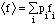.

In this example, the court had the same information about the distribution of l as the actors. If that is true and if C''>0, the court can always do better by imposing a punishment based on expected damage than by imposing a punishment based on actual damage. If, more realistically, the court's information about the actor's ex ante distribution of l is worse than the actor's, punishment based on the court's estimate of expected damage will be less accurate than punishment based on actual damage, since under the latter rule the actor will decide whether to take the action by comparing his gain to his expected punishment calculated using his information about the probability distribution of losses. So the worse the court's information about what the actor knows when he takes the action, the stronger the case for punishment based on actual loss ex post rather than expected loss ex ante.

We have now seen three different reasons why including punishment cost complicates the argument for the simple rule of punishment equal to ex post loss. First, the existence of punishment cost implies that optimal punishment is no longer equal to damage done. Second, if the damage that an act will do is uncertain at the time the act is committed, the schedule of punishment by actual damage done will affect the number of offenses in a complicated way as shown in Equation 9, and the effects must be taken into account in calculating the optimal schedule of punishments. Third, if the cost of punishing an offense increases with increasing size of punishment at an increasing rate (C''(f)>0), a certain punishment f will have a lower cost than a punishment lottery whose expected value is f. There is then an inherent cost advantage to punishment based on the court's estimate of expected damage ex ante instead of actual damage ex post, to be balanced against the disadvantage of an expected punishment based on the court's information rather than the actor's information.

Back to the list of articles.